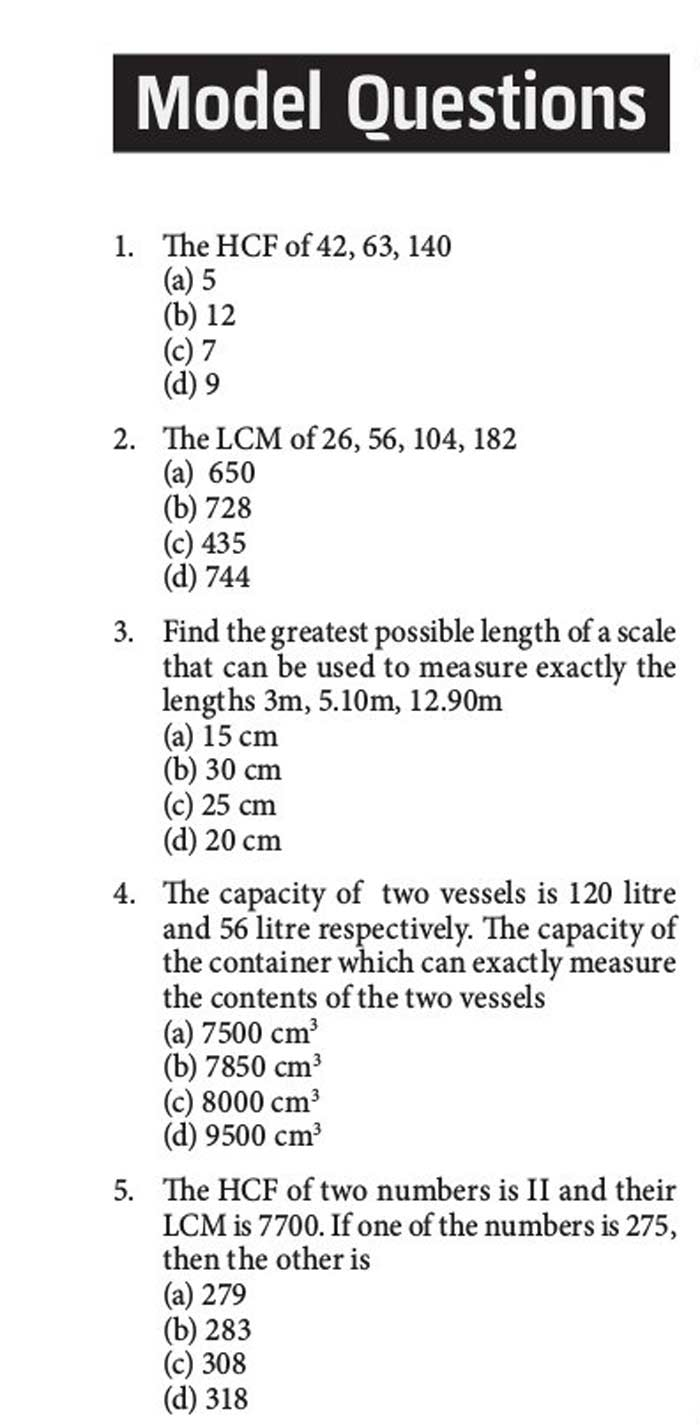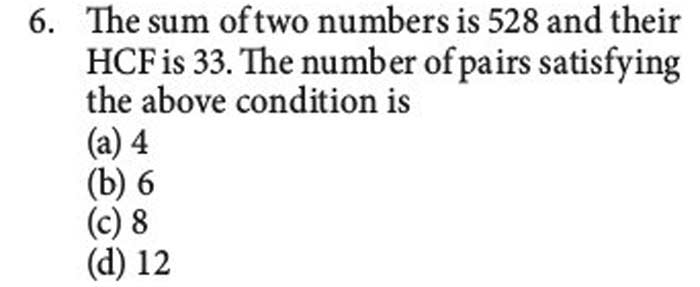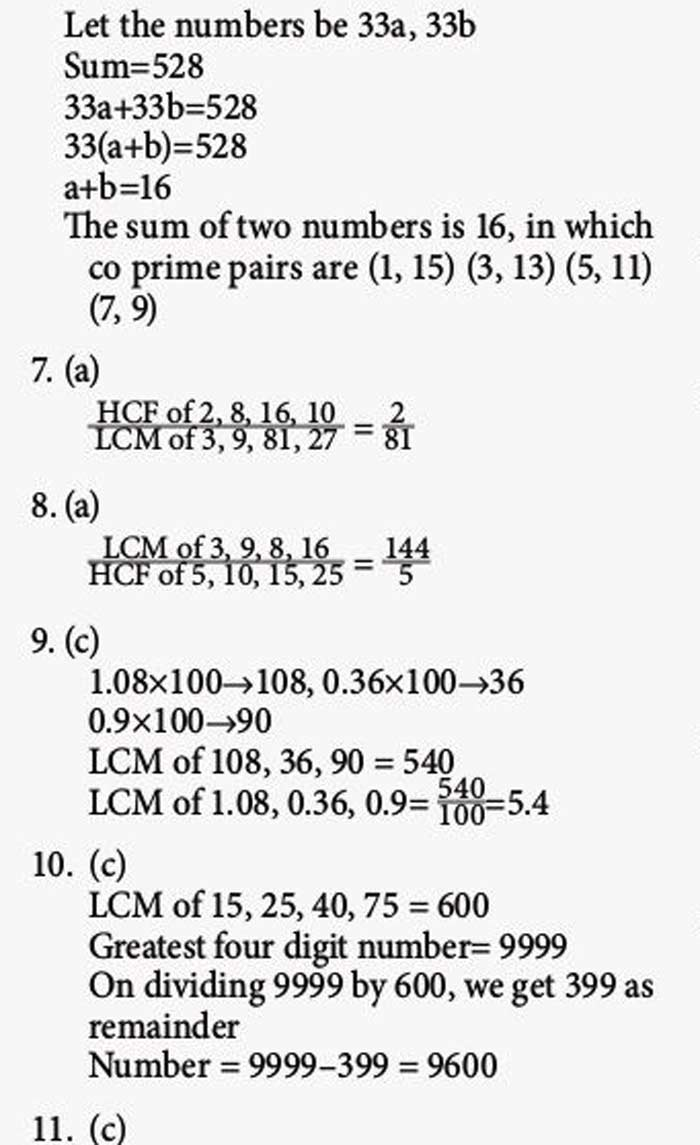## Monday, 29 April 2019

MATHS SOLVED IN STEPS: HCM & LCM -01* MATHS (SOLVED IN STEPS ==> Ratio and Proportion
* MATHS (SOLVED IN STEPS ==> Progressions
* MATHS (SOLVED IN STEPS ==> Percentage
* MATHS (SOLVED IN STEPS ==> Surds and Indices
* MATHS (SOLVED IN STEPS ==> Calendar
* MATHS (SOLVED IN STEPS ==> Square and cube
* MATHS (SOLVED IN STEPS ==> Simplification
* MATHS (SOLVED IN STEPS ==> Problems on Average
* MATHS (SOLVED IN STEPS ==> Profit and Loss
* MATHS (SOLVED IN STEPS ==> Problems on Trains
* MATHS (SOLVED IN STEPS ==>
* MATHS (SOLVED IN STEPS ==>
* MATHS (SOLVED IN STEPS ==>
* MATHS (SOLVED IN STEPS ==>